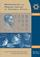Középiskolai Matematikai és Fizikai Lapok
Informatika rovattal
 Már regisztráltál? Új vendég vagy?# Angol nyelvű szám, 2002. december

Előző oldalTartalomjegyzékKövetkező oldalMEGRENDELŐLAP

## Preparatory problems for the entrance exam of high school

1. Solve the following equation on the set of real numbers:

$\displaystyle \frac{2x+2}{7}=\frac{(x^2-x-6)(x+1)}{x^2+2x-3}.$

2. For what positive integers a is the value of the following expression also an integer?

$\displaystyle \left(\frac{a+1}{1-a}+\frac{a-1}{a+1}-\frac{4a^2}{a^2-1}\right) :\left(\frac{2}{a^3+a^2}-\frac{2-2a+2a^2}{a^2}\right)$

3. Given that the second coordinates of the points A(1,a), B(3,b), C(4,c) are

$\displaystyle a=-\frac{\sin39^\circ+\sin13^\circ}{\sin26^\circ\cdot\cos13^\circ},\qquad b=\sqrt{10^{2+\log_{10}25}},\qquad c= \left(\frac{1}{\sqrt{5}-2}\right)^3-\left( \frac{1}{\sqrt{5}+2}\right)^3$

determine whether the three points are collinear.

4. What is more favourable:

I. If the bank pays 20% annual interest, and the inflation rate is 15% per year, or
II. if the bank pays 12% annual interest, and the inflation rate is 7% per year?

5. The first four terms of an arithmetic progression of integers are a1,a2,a3,a4. Show that 1.a12+ 2.a22+3.a32+ 4.a42 can be expressed as the sum of two perfect squares.

6. In an acute triangle ABC, the circle of diameter AC intersects the line of the altitude from B at the points D and E, and the circle of diameter AB intersects the line of the altitude from C at the points F and G. Show that the points D, E, F, G lie on a circle.

7. The base of a right pyramid is a triangle ABC, the lengths of the sides are AB=21 cm, BC=20 cm and CA=13 cm. A', B', C' are points on the corresponding lateral edges, such that AA'=5 cm, BB'=25 cm and CC'=4 cm. Find the angle of the planes of triangle A'B'C' and triangle ABC.

8. Let f(x)= 2x6- 3x4+x2. Prove that f(sin $\displaystyle \alpha$)+f(cos $\displaystyle \alpha$)=0.